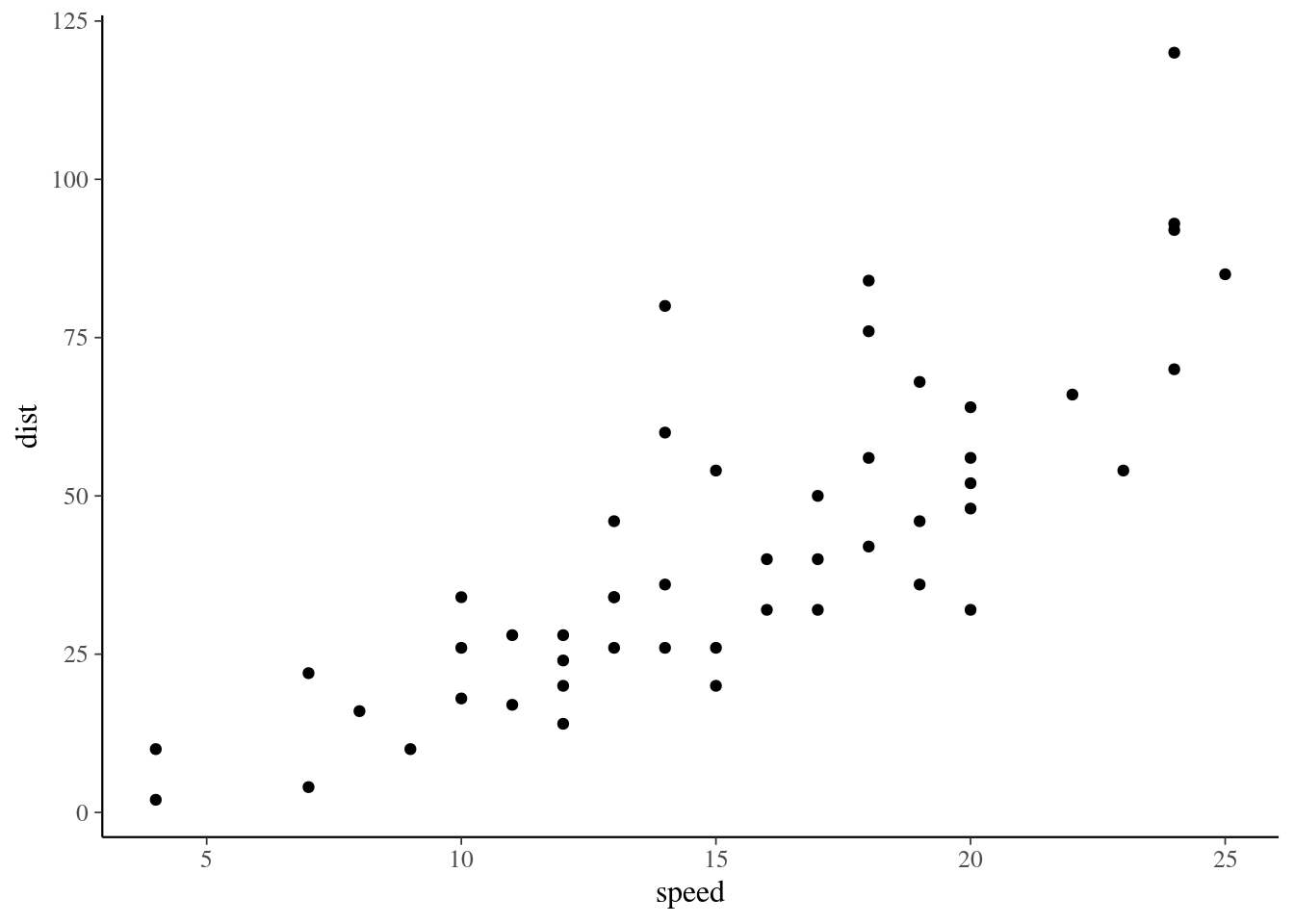## Introduction

The Bayesian paradigm has become increasingly popular, but is still not as widespread as “classical” statistical methods (e.g. maximum likelihood estimation, null hypothesis significance testing, etc.). One reason for this disparity is the somewhat steep learning curve for Bayesian statistical software. The rstanarm package aims to address this gap by allowing R users to fit common Bayesian regression models using an interface very similar to standard functions R functions such as lm() and glm(). In this seminar we will provide an introduction to Bayesian inference and demonstrate how to fit several basic models using rstanarm.

### Bayesian Inference

Bayesian inference provides a principled method of combining prior information concerning parameters (such as regression coefficients) with observed outcome and covariate data based on Bayes’ Theorem: $\begin{equation} \underbrace{p(\theta|X, y)}_\text{posterior distribution}=\frac{\overbrace{p(\theta)}^\text{prior} \, \overbrace{p(y|X, \theta)}^\text{likelihood} }{ \underbrace{\int p(\theta)p(y|X, \theta)\,d\theta}_\text{marginal likelihood}}\\ \end{equation}$ The likelihood (aka sampling distribution) $$p(y|X, \theta)$$ represents the contribution from the observed data and $$p(\theta)$$ represents prior information (or absence of information) about the parameters. The combination of these two components yields the posterior probability $$p(\theta|y, X)$$ which represents an updated distribution for the parameters conditional on the observed data. Note that if $$y$$ is fixed the marginal likelihood is constant with respect to $$\theta$$, so we can write the equation above in terms of the unnormalized posterior distribution: $$p(\theta|X, y) \propto p(\theta) p(y|X, \theta)$$. Performing inference for regression models in a Bayesian framework has several advantages:

• Can formally incorporate information from multiple sources including prior information if available

• Inference is conditional on actual observed data, rather than data that could have been observed

• Can answer inferential questions using interpretable posterior probability statements rather than hypothesis tests and p-values which are dominant in the frequentist paradigm (e.g., “Given our model, prior knowledge, and the observed data, there is a 92% probability that the drug is effective”)

• All inference proceeds directly from the posterior distribution

### Stan, rstan, and rstanarm

Stan is a general purpose probabilistic programming language for Bayesian statistical inference. It has interfaces for many popular data analysis languages including Python, MATLAB, Julia, and Stata. The R interface for Stan is called rstan and rstanarm is a front-end to rstan that allows regression models to be fit using a standard R regression model interface.

# install.packages("rstanarm") # may take a while
library(rstan)
library(rstanarm)
library(ggplot2)
library(bayesplot)

# this option uses multiple cores if they're available
options(mc.cores = parallel::detectCores()) 

### Steps for Bayesian inference

1. Specify the probability model. As discussed above the model has two parts, a likelihood for the observed data and a prior distribution. In practice, the form of the likelihood is usually based on the outcome type and is often the same as a frequentist analysis. Specifying the prior distribution can be more involved, but rstanarm includes default priors that work well in many cases.

2. Draw samples from the posterior distribution. Once the model is specified, we need to get an updated distribution of the parameters conditional on the observed data. Conceptually, this step is simple, but in practice it can be quite complicated. By default, Stan uses a method called Markov Chain Monte Carlo (MCMC) to get these samples.

3. Evaluate the model. There are several important checks that are necessary to ensure that there are no problems with the MCMC procedure used to get samples or the posterior distribution.

4. Perform inference. Once we have verified that everything looks good with our model and MCMC sampling, we can use the samples to perform inference on any quantity involving the posterior parameter distribution.

## Linear Regression model with continuous outcome

Let’s see how to apply these steps for a linear regression model. We’ll use the simple cars dataset which gives the speed of 50 cars along with the corresponding stopping distances.

qplot(speed, dist, data=cars)1. Specify the probability model. For this example, assume the usual linear regression model where each outcome observation (dist) is conditionally normal given the covariate (speed) with independent errors, $$\text{dist} = \beta_0 + \beta_1\cdot \text{speed} + \varepsilon$$, $$\, \varepsilon \sim N(0,\sigma^2)$$. This implies the posterior will have 3 parameters, $$\beta_0$$, $$\beta_1$$ and $$\sigma^2$$. We will let rstanarm use the default priors for now to complete the probability model specification.

2. Draw samples from the posterior distribution. We can use the rstanarm function stan_glm() to draw samples from the posterior using the model above. Comparing the documentation for the stan_glm() function and the glm() function in base R, we can see the main arguments are identical.

stan_glm(formula, family = gaussian(), data, weights, subset,
na.action = NULL, offset = NULL, model = TRUE, x = FALSE,
y = TRUE, contrasts = NULL, ..., prior = normal(),
prior_intercept = normal(), prior_aux = exponential(),
prior_PD = FALSE, algorithm = c("sampling", "optimizing",
"meanfield", "fullrank"), mean_PPD = algorithm != "optimizing",
adapt_delta = NULL, QR = FALSE, sparse = FALSE)

glm(formula, family = gaussian, data, weights, subset,
na.action, start = NULL, etastart, mustart, offset,
control = list(...), model = TRUE, method = "glm.fit",
x = FALSE, y = TRUE, singular.ok = TRUE, contrasts = NULL, ...)

The actual model can be fit with a single line of code.

glm_post1 <- stan_glm(dist~speed, data=cars, family=gaussian)
1. Evaluate the model. Two common checks for the MCMC sampler are trace plots and $$\hat{R}$$. We use the function stan_trace() to draw the trace plots which show sequential draws from the posterior distribution. Ideally we want the chains in each trace plot to be stable (centered around one value) and well-mixed (all chains are overlapping around the same value).
stan_trace(glm_post1, pars=c("(Intercept)","speed","sigma"))